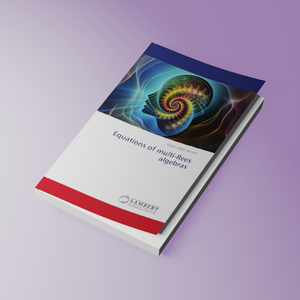# Equations of multi-Rees algebras

Regular price
€39,90
Sale price
€39,90
Regular price
Sold out
Unit price
per
Shipping calculated at checkout.

Summary:

Describing defining equations of Rees algebra is a central problem in commutative algebra. More generally, given a family of ideals, one would like to describe the equations of the multi-Rees algebra of these ideals. Indeed, the multi-Rees algebra in question is simply the Rees algebra of the module. There is little work on the defining equations of the multi-Rees algebra compared to the ordinary Rees algebra. Another motivation for investigating the multi-Rees algebra is an illustration of the theory of Rees algebra of modules. In this work first, we describe defining equations of multi-Rees algebras of powers of an ideal of linear type. In the second part we describe defining equations of multi-Rees algebras of a family of ideals, where these ideals are generated by subsets of a regular sequence.

Author:

Biographie:

Babak Jabbar Nezhad received his PhD in mathematics from the University of Arkansas at Fayetteville in August 2018. His research area is commutative algebra concentrating on problems related to Rees algebras of modules. Also, he is working on other problems in commutative algebra such as determinantal ideals.

Number of Pages:

60

Book language:

English

Published On:

2020-06-29

ISBN:

978-620-2-67296-2

Publishing House: